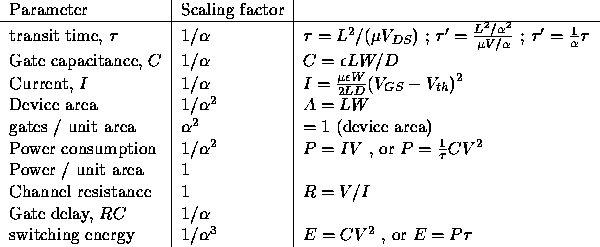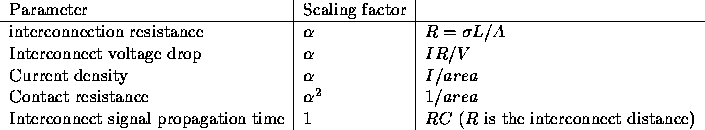Scaling effects in MOS devices

We will consider the effect of applying a unitless scale factor ofto the geometric parameters of a MOS transistor. In order to allow the technology to ``scale'', one constraint we can apply is to insist that the total power consumed per unit area remain constant. (This means that, as the density of circuitry in a technology increases, the amount of cooling required for the circuits will remain constant --- if this was allowed to increase, after some point, the circuits would melt!)

If we scale the following parameters in the way indicated, the power consumed per unit area will remain constant:We can see from the relations previously developed that the following parameters scale in the manner indicated:The following parameters also scale as shown, but the effects are undesirable:root
Mon Mar 27 21:13:11 NST 1995